# Tangent + right triangle - math problems

#### Number of problems found: 143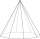You need to make a pad in the shape of a regular octagon with a side length of 4 cm. What is the minimum diameter of the circle-shaped semi-finished product from which we make the pad, and what will be the percentage of waste? (Round the results to 1 deci
• Touch circle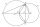Point A has a distance IA, kl = 10 cm from a circle k with radius r = 4 cm and center S. Calculate: a) the distance of point A from the point of contact T if the tangent to the circle is drawn from point A b) the distance of the contact point T from the l
• TrapezoidsIn the isosceles trapezoid ABCD we know: AB||CD, |CD| = c = 8 cm, height h = 7 cm, |∠CAB| = 35°. Find the area of the trapezoid.
• A missile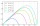A missile is fired with a speed of 100 fps in a direction 30° above the horizontal. Determine the maximum height to which it rises? Fps foot per second.
• Isosceles trapezoidFind the height in an isosceles trapezoid if the area is 520 cm2 and the base a = 25 cm and c = 14 cm. Calculate the interior angles of the trapezoid.
• Sin cos tan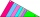In triangle ABC, right-angled at B. Sides/AB/=7cm, /BC/=5cm, /AC/=8.6cm. Find to two decimal places. A. Sine C B. Cosine C C. Tangent C.
• Three pillars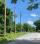On a straight road, three pillars are 6 m high at the same distance of 10 m. At what angle of view does Vlado see each pillar if it is 30 m from the first and his eyes are at 1.8 m high?
• CalculateCalculate the area of triangle ABC, if given by alpha = 49°, beta = 31°, and the height on the c side is 9cm.
• Tower's viewFrom the church tower's view at the height of 65 m, the top of the house can be seen at a depth angle of alpha = 45° and its bottom at a depth angle of beta = 58°. Calculate the height of the house and its distance from the church.
• AircraftFrom the aircraft flying at an altitude of 500m, they observed places A and B (located at the same altitude) in the direction of flight at depth angles alpha = 48° and beta = 35°. What is the distance between places A and B?
• Altitude angle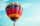In complete winds-free weather, the balloon took off and remained standing exactly above the place from which it took off. It is 250 meters away from us. How high did the balloon fly when we see it at an altitude angle of 25°?
• Base diagonalIn a regular 4-sided pyramid, the side edge forms an angle of 55° with the base's diagonal. The length of the side edge is eight meters. Calculate the surface area and volume of the pyramid.
• Side edgesThe regular 4-sided pyramid has a body height of 2 dm, and the opposite side edges form an angle of 70°. Calculate the surface area and volume of the pyramid.
• Diagonals of a prismThe base of the square prism is a rectangle with dimensions of 3 dm and 4 dm. The height of the prism is 1 m. Find out the angle between the body diagonal with the diagonal of the base.
• The body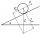The body slides down an inclined plane forming an angle α = π / 4 = 45° under the action of a horizontal plane under the effect of friction forces with acceleration a = 2.4 m/s ^ 2. At what angle β must the plane be inclined so that the body slides on it
• Truncated pyramidFind the volume of a regular 4-sided truncated pyramid if a1 = 14 cm, a2 = 8 cm and the angle that the side wall with the base is 42 degrees
• The staircaseThe staircase has a total height of 3.6 m and forms an angle of 26° with the horizontal. Calculate the length of the whole staircase.
• Space diagonal anglesCalculate the angle between the body diagonal and the side edge c of the block with dimensions: a = 28cm, b = 45cm and c = 73cm. Then, find the angle between the body diagonal and the plane of the base ABCD.The regular quadrilateral pyramid has a base edge a = 1.56 dm and a height h = 2.05 dm. Calculate: a) the deviation angle of the sidewall plane from the base plane b) deviation angle of the side edge from the plane of the baseThe height of a regular quadrilateral prism is v = 10 cm, the deviation of the body diagonal from the base is 60°. Determine the length of the base edges, the surface, and the volume of the prism.